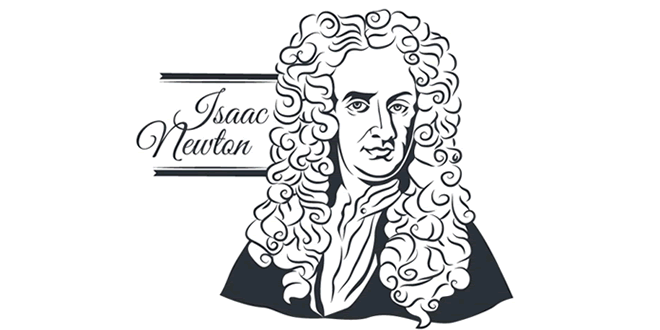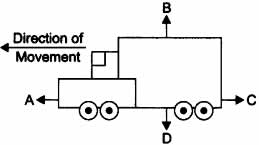Thursday , July 7 2022# 9th Class (CBSE) Science: Force and Laws of Motion

#### Question: Define force.

Answer: It is a push or pull on an object that produces acceleration in the body on which it acts.

#### Question: What is S.I. unit of force?

Answer: S.I. unit of force is Newton.

#### Question: Define one Newton.

Answer: A force of one Newton produces an acceleration of 1 m/s2 on an object of mass 1 kg. 1 N = 1 kg m/s2

#### Question: What is balanced force?

Answer: When forces acting on a body from the opposite direction do not change the state of rest or of motion of an object, such forces are called balanced forces.

#### Question: What is frictional force?

Answer: The force that always opposes the motion of object is called force of friction.

#### Question: What is inertia?

Answer: The natural tendency of an object to resist a change in their state of rest or of uniform motion is called inertia.

#### Question: State Newton’s first law of motion.

Answer: An object remains in a state of rest or of uniform motion in a straight line unless acted upon by an external unbalanced force.

#### Question: State Newton’s second law of motion.

Answer: The rate of change of momentum of an object is proportional to the applied unbalanced force in the direction of the force.

#### Question: What is momentum?

Answer: The momentum of an object is the product of its mass and velocity and has the same direction as that of the velocity. The S. I. unit is kg m/s. (p = mv)

#### Question: State Newton’s III law of motion.

Answer: To every action, there is an equal and opposite reaction and they act on two different bodies.

#### Question: Which will have more inertia a body of mass 10 kg or a body of mass 20 kg?

Answer: A body of mass 20 kg will have more inertia.

#### Question: Name the factor on which the inertia of the body depends.

Answer: Inertia of a body depends upon the mass of the body.

#### Question: Name two factors which determine the momentum of a body.

Answer: Two factors on which momentum of a body depend is mass and velocity. Momentum is directly proportional to the mass and velocity of the body.

#### Question: What decides the rate of change of momentum of an object?

Answer: The rate of change of momentum of an object is proportional to the applied unbalanced force in the direction of force.

#### Question: The diagram shows a moving truck. Forces A, B, C and D are acting on the truck. Name the type of forces acting on a truck.Answer: The forces A, B, C and D acting on the truck are:

A → driving force

B → reacting force

C → frictional force

D → weight/gravitational force

## Sources of Energy: 10th Science Chapter 14

Class: 10th Class Subject: Science Chapter: Chapter 14: Sources of Energy Quiz: – Questions MCQs: …